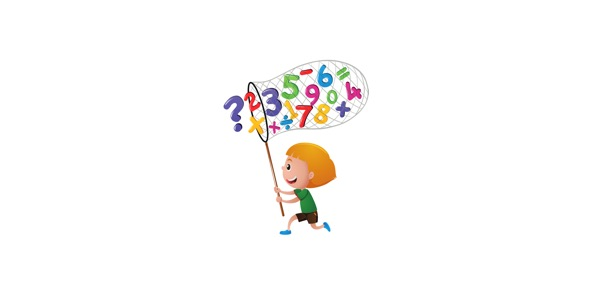# 6th Grade Chapter 7 Section 1 Quiz

16 Questions | Attempts: 146
ShareSettingsChapter 7 Section 1- What is work?

• 1.
When a force produces motion parallel to the direction of the force, ___________ is done in the scientific sense.
• A.

Power

• B.

Work

• C.

Motion

• 2.
When force is at a ___________ angle to the direction of motion, the force does not work.
• A.

Right

• B.

Acute

• C.

Obtuse

• 3.
When yo pick up a book bag, you do __________________.
• A.

Negative work

• B.

Positive work

• 4.
Friction does ______________ work on a sled.
• A.

Positive

• B.

Negative

• 5.
When you pull a sled, you do _______________ work on the sled.
• A.

Positive

• B.

Negative

• 6.
When the motion is in the direction of the force, ______________ work is done.
• A.

Positive

• B.

Negative

• 7.
When the motion is opposite to the direction of the force, ____________ work is done.
• A.

Positive

• B.

Negative

• 8.
Carrying a ball off the field is ______________.
• A.

Positive work

• B.

Negative work

• C.

No work

• 9.
What is the formula for work?
• A.

P = W/t

• B.

W = Fd

• C.

R = d/t

• 10.
Work is measured in ______________.
• A.

Joules - J

• B.

Meters - m

• C.

Seconds - s

• D.

Kilograms - kg

• 11.
1 J is the same as ___________ .
• A.

1 N

• B.

1 kg * m2/s2

• C.

1 kg * m/s

• 12.
If you walk 1000 m using about 100 N of force to push a lawn mower forward.  How much work is done?
• A.

10 N * m

• B.

100,000 N

• C.

100,000 J

• 13.
What describes the rate at which work is being done?
• A.

Force

• B.

Power

• C.

Speed

• 14.
What is the formula for power?
• A.

P = W/t

• B.

W = Fd

• C.

R = d/t

• D.

R = W/t

• 15.
Power is measured in what unit?
• A.

Volt - V

• B.

Joules - J

• C.

Watt - W

• 16.
1 W = ?
• A.

1 J/s

• B.

1 J

• C.

1 kg * m/s

## Related TopicsBack to top
×

Wait!
Here's an interesting quiz for you.